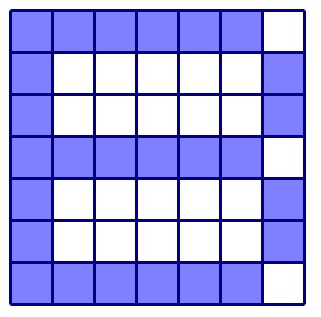# B is for Brilliant

Probability Level 5

In each cell of the following $7\times7$ square table, write one of the numbers $1,2,3,4,5,6,7$ such that the numbers in each row and each column are distinct. What is the greatest possible value of the sum of the numbers written in the blue squares?×